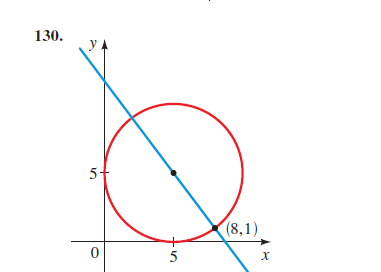# The equation for the circle and line in the given figure.### Precalculus: Mathematics for Calcu...

6th Edition
Stewart + 5 others
Publisher: Cengage Learning
ISBN: 9780840068071### Precalculus: Mathematics for Calcu...

6th Edition
Stewart + 5 others
Publisher: Cengage Learning
ISBN: 9780840068071

#### Solutions

Chapter 1, Problem 130RE
To determine

## To calculate:The equation for the circle and line in the given figure.

Expert Solution

The equation for the circle and line in the figure are x2+y210x10y+25=0 and 4x3y+35=0 respectively.

### Explanation of Solution

Given information:The point P(8,1) lying on the tangent to the circle with center (h,k)=(5,5)

Formula used:

For a given circle with center (h,k) and radius r the equation is given as:

(xh)2+(yk)2=r2

This is referred to as the Standard form for the equation of a given circle.

The general equation for the circle is:

x2+y2+2gx+2fy+c=0

Where (g,f) denotes the center of the circle and radius is given by r=g2+f2c

The distance D between two points say (x1,y1) and (x2,y2) is given as:

D=(x2x1)2+(y2y1)2

Slope m of the line passing through two points in general say P1=(x1,y1) and P2=(x2,y2) is:

m=y2y1x2x1

Slope-intercept equation for a given line which has slope as m and y −intercept as b is:

y=mx+b

Two-intercept equation for a given line which has x -intercept as a and y −intercept as b is:

xa+yb=1

When two lines are perpendicular then the product of their slopes is zero that is m1.m2=1

When two lines are parallel then their slope are equal that is m1=m2

Calculation:

From the given figure it is clear that center of the circle is (h,k)=(5,5)

Recall for a given circle with center (h,k) and radius r the equation is given as:

(xh)2+(yk)2=r2

Therefore, replacing the values we get:

(x5)2+(y5)2=r2 (1)

Now to find radius r which is the distance from the center (h,k)=(5,5) to the point P(8,1)

Recall, the distance D between two points say (x1,y1) and (x2,y2) is given as:

D=(x2x1)2+(y2y1)2

Before applying distance formula the following must be known:

x1=5y1=5x2=8y2=1

Therefore,

D=r=(85)2+(15)2=9+16=25=5

Put this value in (1)

(x5)2+(y5)2=52(x5)2+(y5)2=25

Recall the identities:

(a+b)2=a2+2ab+b2(ab)2=a22ab+b2

Use this to get:

x2+2510x+y2+2510y=25x2+y210x10y+25=0

Hence, the equation for the given circle is x2+y210x10y+25=0

Now for the equation of line:

Recall, slope m of the line passing through two points in general say P1=(x1,y1) and P2=(x2,y2) is:

m=y2y1x2x1

As it is known: P1=(5,5) and P2=(8,1)

x1=5y1=5x2=8y2=1

Therefore, slope of line is:

m=1585m=43

Slope of line is m=43

And it passes through P1=(5,5) that is x=5y=5

Put these values in the general equation for the line which is y=mx+b (2)

Which gives:

5=43(5)+bb=5+203b=15+203b=353

Now put m=43 and b=353 in (2) to get:

y=43x+3533y=4x+354x3y+35=0

Thus, required equation of line is 4x3y+35=0

### Have a homework question?

Subscribe to bartleby learn! Ask subject matter experts 30 homework questions each month. Plus, you’ll have access to millions of step-by-step textbook answers!